# Galois group

(diff) ← Older revision | Latest revision (diff) | Newer revision → (diff)
The automorphism group of a Galois extensionof a field, i.e. the group of all automorphisms of the fieldleaving the elements of the subfieldfixed. The group is denoted by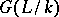or by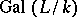. The field of invariants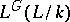coincides with the field. Ifis the splitting field of a polynomialover, the Galois groupis also called the Galois group of the polynomial. These groups are important in the Galois theory of algebraic equations. The computation of the Galois groups for extensions of algebraic number fields is one of the fundamental tasks of algebraic number theory. Finding Galois extensions with an Abelian Galois group (Abelian extensions) is a part of class field theory. Galois groups of algebraic function fields are also a subject of algebraic geometry.
Letbe a field and letbe a finite subgroup of the automorphism group of;will then be a Galois extension of the field of invariants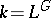, and the Galois group of this extension is isomorphic to; moreover, the degree of the extension,, is equal to the order of.
The fundamental result on Galois groups is the following theorem, which is sometimes called the main theorem on Galois extensions or the theorem on Galois correspondence. Ifis a Galois extension of finite degree of a field, then there exists a one-to-one correspondence between all subgroupsof the Galois groupand all subfieldsofthat contain, and theandcorresponding to each other are such thatis the field of invariants ofandis the Galois group of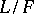(cf. Galois correspondence). This theorem has numerous analogues in many mathematical theories, and can be generalized to extensions of infinite degree (cf. Galois topological group). There exists a generalization of the concept of a Galois group to extensions of arbitrary commutative rings, schemes (cf. Fundamental group), and also to the case of extensions of skew-fields.